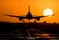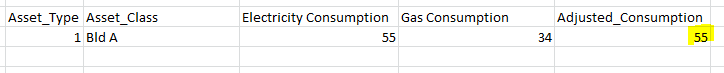cancel
Showing results for
Did you mean:Frequent Visitor

## Summing Column on Multiple Category

Hello All,

I have this tableI already have a Tabular Model created to be used in Power BI. I was wondering how to calculated the adjusted consumption using DAX based on its formula shown below the table?

Thanks,

1 ACCEPTED SOLUTIONSuper User

Hi @baw556

Do you want it across all asset types and classes? If so, place tis measure in a card visual:

```Measure =
VAR Constant_ = 4.3456
RETURN
CALCULATE ( SUM ( Table1[Consumption] ), Table1[Fuel] = "Electricity" )
+ CALCULATE ( SUM ( Table1[Consumption] ), Table1[Fuel] = "Gas" ) * Constant_
```

Please mark the question solved when done and consider giving kudos if posts are helpful.

Cheers3 REPLIES 3Super User

Hi @baw556

Do you want it across all asset types and classes? If so, place tis measure in a card visual:

```Measure =
VAR Constant_ = 4.3456
RETURN
CALCULATE ( SUM ( Table1[Consumption] ), Table1[Fuel] = "Electricity" )
+ CALCULATE ( SUM ( Table1[Consumption] ), Table1[Fuel] = "Gas" ) * Constant_
```

Please mark the question solved when done and consider giving kudos if posts are helpful.

CheersFrequent Visitor

Yes, I wanted to aggregate accross asset type and class.

When I appplied the formula, it only aggregates the valus for Electricity and doesn't include the secod part of the formula (Gas cons. * constant)

Any thoughts?

Thanks,

Baw556Frequent Visitor

The desired result from below should be Adjusted_consumption approx. 203. However, using the formula above, I'm still getting 55.